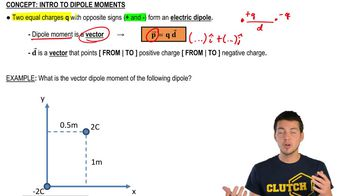Start typing, then use the up and down arrows to select an option from the list.
3:52 minutes
Problem 21b
Textbook Question

# Point charges q1 = -4.5 nC and q2 = +4.5 nC are separated by 3.1 mm, forming an electric dipole. (b) The charges are in a uniform electric field whose direction makes an angle of 36.9° with the line connecting the charges. What is the magnitude of this field if the torque exerted on the dipole has magnitude 7.2 * 10^-9 N•m?Verified Solution
This video solution was recommended by our tutors as helpful for the problem above.
58views